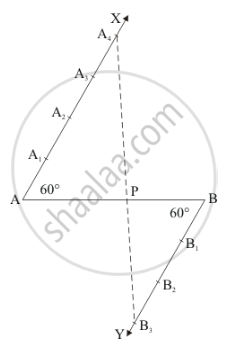Share

# Divide a Line Segment of Length 9 Cm Internally in the Ratio 4 : 3. Also, Give Justification of the Construction. - CBSE Class 10 - Mathematics

#### Question

Divide a line segment of length 9 cm internally in the ratio 4 : 3. Also, give justification of the construction.

#### Solution

Given that

Determine a point which divides a line segment of length 9 cm internally in the ratio of 4:3.

We follow the following steps to construct the givenStep of construction

Step: I- First of all we draw a line segment AB = 9cm.

Step: II- We draw a ray AX making an acute angle ∠BAX = 60° with AB.

Step: III- Draw a ray BY parallel to AX by making an acute angle ∠ABY = ∠BAX.

Step IV- Mark of two points A1, A2, A3, A4 on AX and three points  B1, B2, B3 on BY in such a way that AA1 = A1A2 = A2A3 = A3A4 = BB1 = B1B2 = B2B3.

Step: V- Joins A4B3  and this line intersects AB at a point P.

Thus, P is the point dividing AB internally in the ratio of 4:3

Justification:

In ΔAA4P and ΔBB3P we have

∠A4AP = ∠PBB3          [∠ABY = ∠BAX]

And ∠APA4 = ∠BPB3    [Vertically opposite angle]

So, AA similarity criterion, we have

ΔAA4P ≈ ΔBB3P

(A A_4)/(BB_3)=(AP)/(BP)

(AP)/(BP)=4/3

Is there an error in this question or solution?

#### APPEARS IN

Solution Divide a Line Segment of Length 9 Cm Internally in the Ratio 4 : 3. Also, Give Justification of the Construction. Concept: Division of a Line Segment.
S